Sunday, October 1, 2023
HomeWINDOWSExcel not auto calculating formulas

# Excel not auto calculating formulas [Fix]

-

Excel is an advanced tool when calculating formulas for statistics and mathematics purposes, but what if when you try to make changes to your data, there is no auto-calculation of the formula? One of the reasons for the issue is the auto-calculation feature is disabled. In this tutorial, we will explain how to fix the issue when Excel is not auto calculating formulas.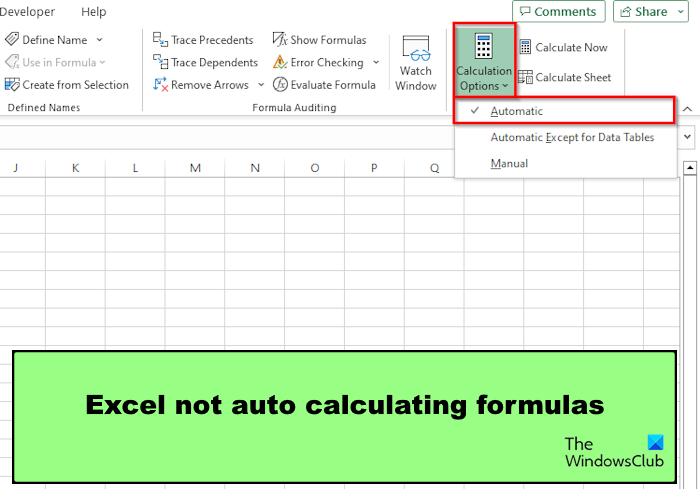## Excel not auto calculating formulas

Follow the suggestions below to fix the issue where Excel is not calculating formulas:

1. Enable the Auto-Calculate option.
2. Disable Show Formula option.
3. Check the cell format.
4. Check if the formula is entered correctly.
5. Check for Circular References in the spreadsheet.

#### 1] Enable the Auto-Calculate option.

There are two methods that you can use to enable the auto-calculate option in Excel: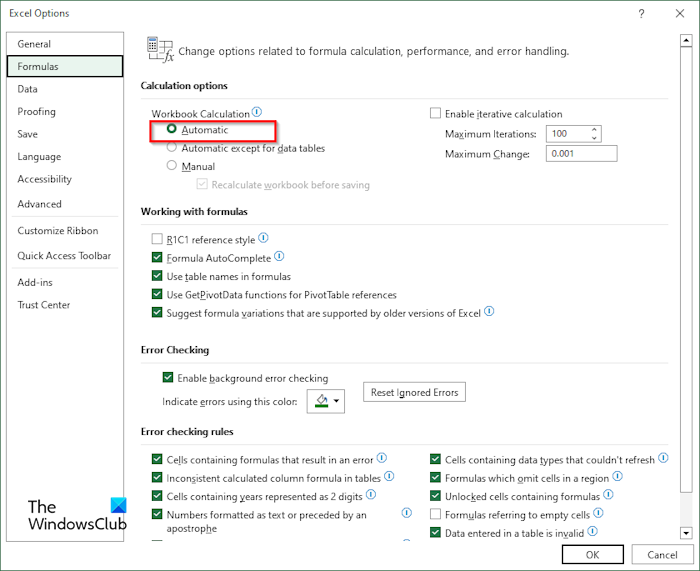Method 1: Go to the File tab.

On the backstage view, click Options.

An Excel options dialog box will open.

Select the Formula tab on the left pane.

Under the Calculation Options section, check Automatic, then click OK.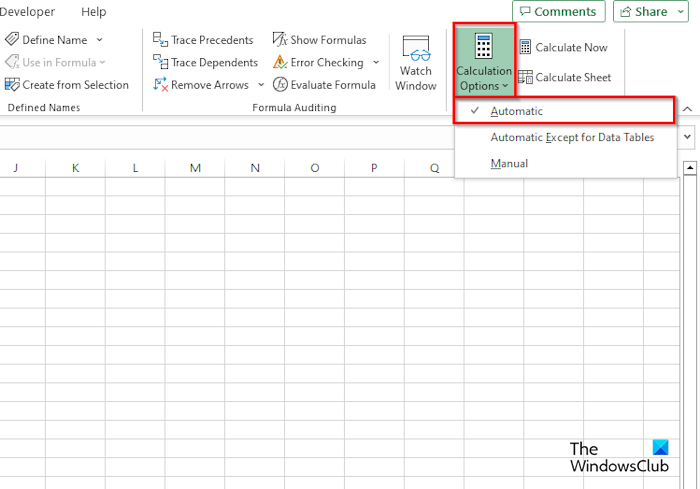Method 2: On the Formulas tab, click the Calculation Options button, then select Automatic from the menu.

#### 2] Disable Show Formula option

If the spreadsheet shows the formula instead of the result, this means that the Show Formulas feature is turned on; you must turn it off; follow the steps below.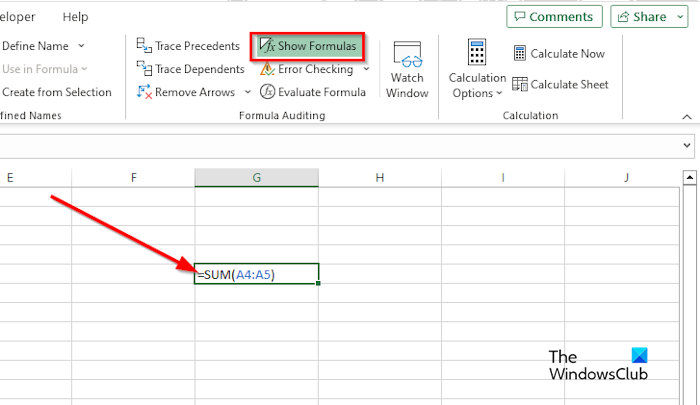On the Formulas tab, click the Show Formulas button in the Formula Auditing group. If the Show Formulas button is highlighted, it is turned on; If it is not highlighted, it is off.

#### 3] Check the cell format

If the formula format is Text, Excel will find it difficult to auto calculate the formula. Change the formula format to General instead. Follow the steps below to change the format from Text to General.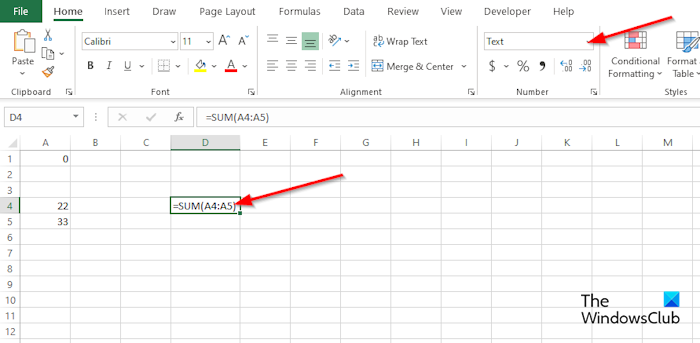On the Home tab in the Number group, check in the list box if the format for the spreadsheet is in Text; if so, click the drop-down arrow and select General from the menu.

#### 4] Check if the formula is entered correctly

If your formula is entered incorrectly, Excel will not auto calculate. The formula will not calculate due to a space entered before the equal sign or an apostrophe is entered as a part of the formula, which stores the formula as text.

#### 5] Check for Circular References in the spreadsheet

Circular Reference occurs whenever a formula refers to its own cell; it is an error. If you want to check for Circular References in your spreadsheet, follow the steps below: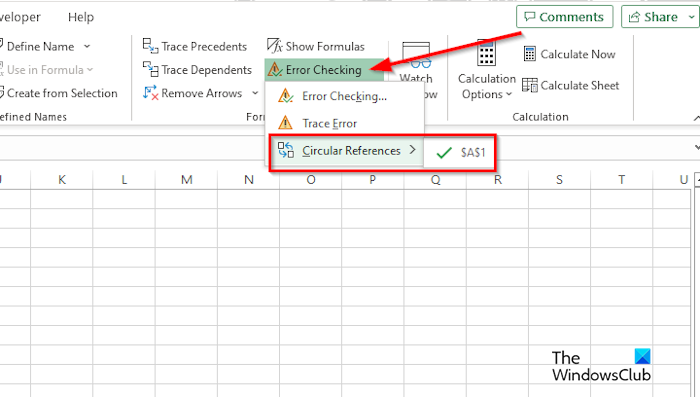Go to the Formulas tab, click the Error Checking list box in the Formula Auditing group, then hover the cursor over Circular Reference, and it will display the error located in your spreadsheet.

### How do I fix formulas in Excel?

In Excel, you can fix an inconsistent formula. Follow the solutions below to do so:

• Click the cell that contains the error.
• Click the Formulas tab, then click the Trace Precedent button.
• Compare the blue arrows or ranges in blue, then correct the problems with the inconsistent formula.
• Click the Remove arrow button in the Formula Auditing group.

### Which calculation mode is enabled by default in Excel workbooks?

The calculation mode that is the default in Excel is Automatic. The Automatic mode allows your calculations or formulas to auto calculate. In Microsoft Excel, there are two modes, namely Manual and Automatic.